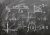# Computational Number Theory and Algebra

Free!

language English Optional, paid
Category:

Algebra plays an important role in both finding algorithms, and understanding the limitations of computation. This course will focus on some of the fundamental algebraic concepts that arise in computation, and the algebraic algorithms that have applications in real life. The course will cover the problems of fast integer (or polynomial) multiplication (or factoring), fast matrix multiplication, primality testing, computing discrete logarithm, error-correcting codes, lattice- based cryptography, etc. The course intends to introduce both basic concepts and practical applications.

COURSE LAYOUT

Week 1:Outline. Notation. Background.
Week 2:GCD. Chinese remaindering. Fast polynomial multiplication.
Week 3: Fast integer multiplication. Fast integer division. Fast gcd.
Week 4:Fast matrix multiplication. Tensor rank.
Week 5:Factorization over finite fields.
Week 6:Berlekamp, Cantor-Zassenhaus factoring algorithms.
Week 7: Reed-Solomon code. List decoding. Bivariate polynomial factoring.
Week 8:Kaltofen’s blackbox multivariate factoring.
Week 9:Integral polynomial factoring. LLL algo. Shortest vector in lattice.
Week 10:Lattice-based cryptography.
Week 11: Primality testing. RSA cryptosystem. Diffie-Hellman. Discrete Log.
Week 12:Integer factoring. Pollard, Fermat, Morrison-Brillhart, Quadratic sieve methods.

## User Reviews

0.0 out of 5
0
0
0
0
0

There are no reviews yet.

×